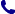• Contact
Choose interesting categories
I agree to receive promotional electronic communication(s).
Unsubscribe
• Institutional Clients
• Delivery
On-line access

# Bookstore

0.00 PLN
Bookshelf (0)

## Oxford IB Diploma Programme: IB Mathematics: analysis and approaches, Higher Level, Print and Enhanced Online Course Book Pack

 Authors Publisher Oxford University Press Year 01/03/2019 Edition First Pages 832 Version mixed media Readership level Adult education Language English ISBN 9780198427162 Categories Mathematics
\$94.39 (with VAT)
419.62 PLN / €89.97 / £78.10
Qty:
Delivery to United States

check shipping prices
Product to order
Delivery 3-4 weeks

## Book description

Featuring a wealth of digital content, this concept-based Print and Enhanced Online Course Book Pack has been developed in cooperation with the IB to provide the most comprehensive support for the new DP Mathematics: analysis and approaches HL syllabus, for first teaching in September 2019. Each Enhanced Online Course Book Pack is made up of one full-colour, print textbook and one online textbook - packed full of investigations, exercises, worksheets, worked solutions and answers, plus assessment preparation support.

Oxford IB Diploma Programme: IB Mathematics: analysis and approaches, Higher Level, Print and Enhanced Online Course Book Pack

From patterns to generalizations: sequences and series
1.1: Sequences, series and sigma notation
1.2: Arithmetic and geometric sequences and series
1.3: Proof
1.4: Counting principles and the binomial theorem
Representing relationships: introducing functions
2.1: Functional relationships
2.2: Special functions and their graphs
2.3: Classification of functions
2.4: Operations with functions
2.5: Function transformations
Expanding the number system: complex numbers
3.2: Complex numbers
3.3: Polynomial equations and inequalities
3.4: The fundamental theorem of algebra
3.5: Solving equations and inequalities
3.6: Solving systems of linear equations
Measuring change: differentiation
4.1: Limits, continuity and convergence
4.2: The derivative of a function
4.3: Differentiation rules
4.4: Graphical interpretation of the derivatives
4.5: Applications of differential calculus
4.6: Implicit differentiation and related rates
Analysing data and quantifying randomness: statistics and probability
5.1: Sampling
5.2: Descriptive statistics
5.3: The justification of statistical techniques
5.4: Correlation, causation and linear regression
Relationships in space: geometry and trigonometry
6.1: The properties of 3D space
6.2: Angles of measure
6.3: Ratios and identities
6.4: Trigonometric functions
6.5: Trigonometric equations
Generalizing relationships: exponents, logarithms and integration
7.1: Integration as antidifferentiation and definite integrals
7.2: Exponents and logarithms
7.3: Derivatives of exponential and logarithmic functions; tangents and normals
7.4: Integration techniques
Modelling changes: more calculus
8.1: Areas and volumes
8.2: Kinematics
8.3: Ordinary differential equations (ODEs)
8.4: Limits revisited
Modelling 3D space: vectors
9.1: Geometrical representation of vectors
9.2: Introduction to vector algebra
9.3: Scalar product and its properties
9.4: Vector equations of a line
9.5: Vector product and properties
9.6: Vector equation of a plane
9.7: Lines, planes and angles
9.8: Application of vectors
Equivalent systems of representation: more complex numbers
10.1: Forms of a complex number
10.2: Operations with complex numbers in polar form
10.3: Powers and roots of complex numbers in polar form
Valid comparisons and informed decisions: probability distributions
11.1: Axiomatic probability systems
11.2: Probability distributions
11.3: Continuous random variables
11.4: Binomial distribution
11.5: The normal distribution
Exploration

## We also recommend books801 777 223